# NCERT Solutions: Comparing Quantities- 1 Notes | Study Mathematics (Maths) Class 7 - Class 7

## Class 7: NCERT Solutions: Comparing Quantities- 1 Notes | Study Mathematics (Maths) Class 7 - Class 7

The document NCERT Solutions: Comparing Quantities- 1 Notes | Study Mathematics (Maths) Class 7 - Class 7 is a part of the Class 7 Course Mathematics (Maths) Class 7.
All you need of Class 7 at this link: Class 7

Exercise 8.1

Question 1:  Find the ratio of:
(a) Rs5 to 50 paise
(b) 15 kg to 210 g
(c) 9 m to 27 cm
(d) 30 days to 36 hours

Answer 1: To find ratios, both quantities should be in same unit.

(a) Rs 5 to 50 paise
⇒  5 x 100 paise to 50 paise    [∴ Rs 1 = 100 paise]
⇒  500 paise to 50 paise

Thus, the ratio is = 500/50 = 10/1 = 10 : 1

(b) 15 kg to 210 g
⇒ 15 x 1000 g to 210 g    [∵ 1kg = 1000 g]
⇒ 15000 g to 210 g
Thus, the ratio is = 15000/210 = 500/7 = 500:7

(c) 9 m to 27 cm
⇒  9 x 100 cm to 27 cm     [∵ 1m = 100 cm]
⇒  900 cm to 27 cm

Thus, the ratio is = 900/27 = 100/3 = 100:3

(d) 30 days to 36 hours
⇒  30 x 24 hours to 36 hours    [∵ 1 day = 24 hours]
⇒  720 hours to 36 hours

Thus, the ratio is = 720/36 = 20/1 = 20:1

Question 2: In a computer lab, there are 3 computers for every 6 students. How many computers will be needed for 24 students?

∵ 6 students need = 3 computers

∵ 1 student needs = 3/6 computers

∵ 24 students need =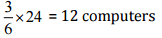Thus, 12 computers will be needed for 24 students.

Question 3: Population of Rajasthan = 570 lakhs and population of U.P. = 1660 lakhs. Area of Rajasthan = 3 lakh km2 and area of U.P. = 2 lakh km2

(i) How many people are there per km2 in both states?

(ii) Which state is less populated?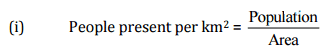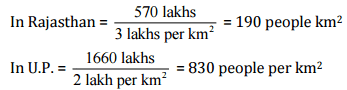(ii) Rajasthan is less populated.

Exercise 8.2

Question 1: Convert the given fractional numbers to percent:

(a) 1/8
(b) 5/4
(c) 3/40
(d) 2/7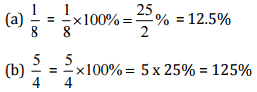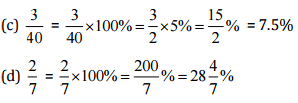Question 2: Convert the given decimal fractions to per cents:

(a) 0.65
(b) 2.1

(c) 0.02
(d) 12.35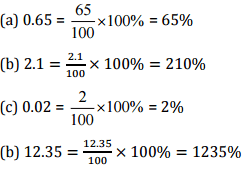Question 3: Estimate what part of the figures is coloured and hence find the percent which is coloured.Answer 3: (i) Coloured part = 1/4

∴ Percent of coloured part =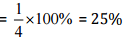(ii) Coloured part = 3/5
∴ Percent of coloured part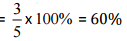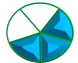(iii) Coloured part = 3/8

∴ Percent of coloured part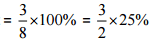= 37.5%

Question 4: Find:

(a) 15% of 250

(b) 1% of 1 hour

(c) 20% of 2500

(d) 75% of 1 kg

Answer 4: (a) 15% of 250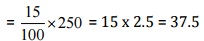(b) 1% of 1 hours = 1% of 60 minutes = 1% of (60 x 60] seconds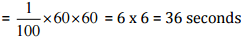(c) 20% of Rs 500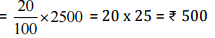(d) 75% of 1 kg = 75% of 1000 g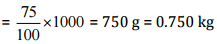Question 5: Find the whole quantity if:

(a) 5% of it is 600

(b) 12% of it is ₹1080

(c) 40% of it is 500 km

(d) 70% of it is 14 minutes

(e) 8% of it is 40 litres

Answer 5: Let the whole quantity be x in given questions: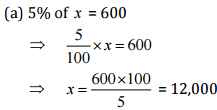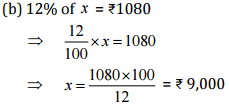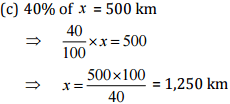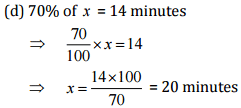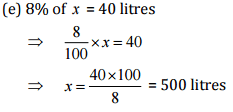Question 6: Convert given per cents to decimal fractions and also to fractions in simplest forms:

(a) 25%

(b) 150%

(c) 20%

(d) 5%

 S. No. Per cents Fractions Simplest form Decimal form (a) 25% 25/100 1/4 0.25 (b) 150% 150/100 3/2 1.5 (c) 20% 20/100 1/5 0.2 (d) 5% 5/100 1/20 0.05

Question 7: In a city, 30% are females, 40% are males and remaining are children. What percent are children?

Answer 7: Given: Percentage of females =30%

Percentage of males = 40%
Total percentage of females and males = 30 + 40 = 70%
Percentage of children = Total percentage - Percentage of males and females
= 100%-70%
= 30%
Hence, 30% are children.

Question 8: Out of 15,000 voters in a constituency, 60% voted. Find the percentage of voters who did not vote. Can you now find how many actually did not vote?

Answer 8: Total voters = 15,000

Percentage of voted candidates = 60%
Percentage of not voted candidates = 100 - 60 = 40%
Actual candidates, who did not vote = 40% of 15000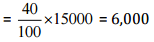Hence, 6,000 candidates did not vote.

Question 9: Meeta saves Rs 400 from her salary. If this is 10% of her salary. What is her salary?

Answer 9: Let Meera's salary be Rs x.

Now, 10% of salary = Rs 400
⇒ 10% of x = Rs 400

Hence, Meera's salary is Rs 4,000.

Question 10: A local cricket team played 20 matches in one season. It won 25% of them. How many matches did they win?

Answer 10: Number of matches played by cricket team = 20

Percentage of won matches = 25%
Total matches won by them = 25% of 20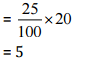Hence, they won 5 matches.

The document NCERT Solutions: Comparing Quantities- 1 Notes | Study Mathematics (Maths) Class 7 - Class 7 is a part of the Class 7 Course Mathematics (Maths) Class 7.
All you need of Class 7 at this link: Class 7Use Code STAYHOME200 and get INR 200 additional OFF

## Mathematics (Maths) Class 7

164 videos|237 docs|45 tests

Track your progress, build streaks, highlight & save important lessons and more!

,

,

,

,

,

,

,

,

,

,

,

,

,

,

,

,

,

,

,

,

,

;